# Linear Time-Invariant (LTI) Simulations in OptSim Circuit

## Introduction

This tutorial is intended to demonstrate the LTI simulation engine of OptSim Circuit. For a more detailed information on the principle of the LTI simulation method, please refer to the User Guide, Chapter 8, Section 8.2. This engine is available only in OptSim Circuit and won’t work in ModeSYS, sample- and blockmode of OptSim.

In a high-density integrated photonic circuit, majority of the photonic components are linear, time-invariant that can be fully characterized in terms of their transfer matrices. Solving such circuits in time-domain can lead to longer simulation times without any gain in simulation accuracy. As we shall see in this case study that employing OptSim Circuit’s LTI simulation engine results in significant speed improvement.

## LTI Simulation Set-Up

The simulation setup can be divided into following steps:

1. Create a new compound component (CC), right-click in the drawing area and choose LTI from its properties
2. Create a circuit using linear photonic components/PDKs
3. Save the CC
4. Create a testbed to simulate the LTI CC Testbed can have non-LTI components and analyzers
5. Specify LTI grid
6. Run simulation

Figure 1 shows step 1.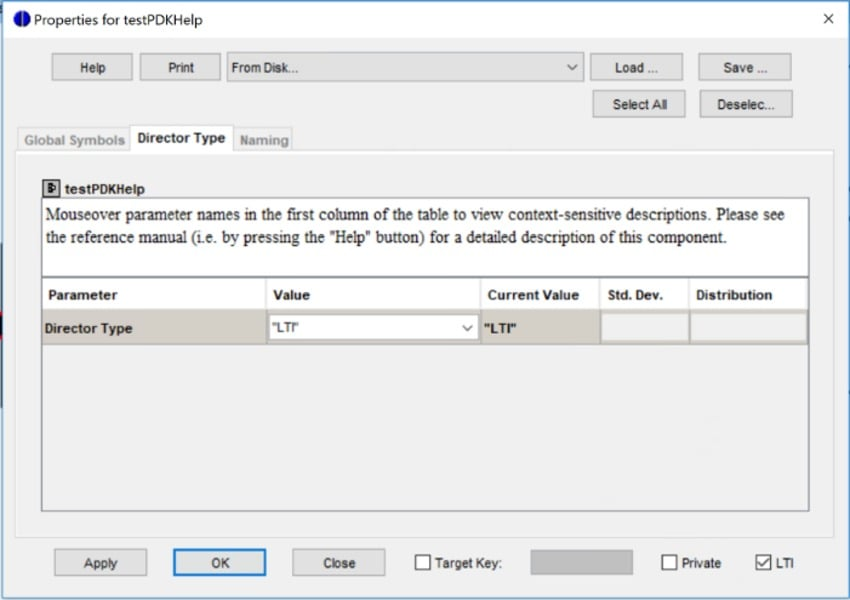Figure 1. Creating a new CC and specifying type as LTI.

## Notes On The LTI Grid

• The LTI Grid represents the frequencies (or wavelengths) at which the LTI S-matrix is calculated
• The grid can be uniformly distributed around the grid center or loaded from file (parameter Grid Specification)
• Uniforms grids are defined by the grid center, bandwidth and number of grid points
• If the incoming signal frequencies are different from the frequencies at which the LTI S-matrix is calculated, interpolation of LTI S-matrix will occur to obtain outputs at the same frequencies of the input signals

## Notes On Grid Grouping

• Grid grouping refers to using two points grouped together for each frequency/wavelength of the main LTI grid
• With grid grouping it’s possible to estimate the phase slope vs frequency/wavelength, and therefore more accurately unwrap the phase and interpolate over larger spacing between grid points
• Grid grouping enables using coarser LTI grids as it removes the requirement of having phase difference between adjacent grid points lower than pi
• f individual S matrices of the elements inside LTI CC don’t use wavelength grouping (and if they should for accurate interpolation), then using wavelength grouping for the entire LTI grid won’t help improve accuracy
• Since grouping doubles the number of frequency points over which LTI S-matrix is calculated, simulation can take longer than without grouping. If grid spacing is close enough so that phase can be accurately interpolated or if the LTI grid and simulation grid exactly match, grouping is not necessary

## A Quick Summary of LTI Grid Setting

The LTI simulation parameters are set in “LTI Options” tab of the simulation run dialog window, shown in Figure 2.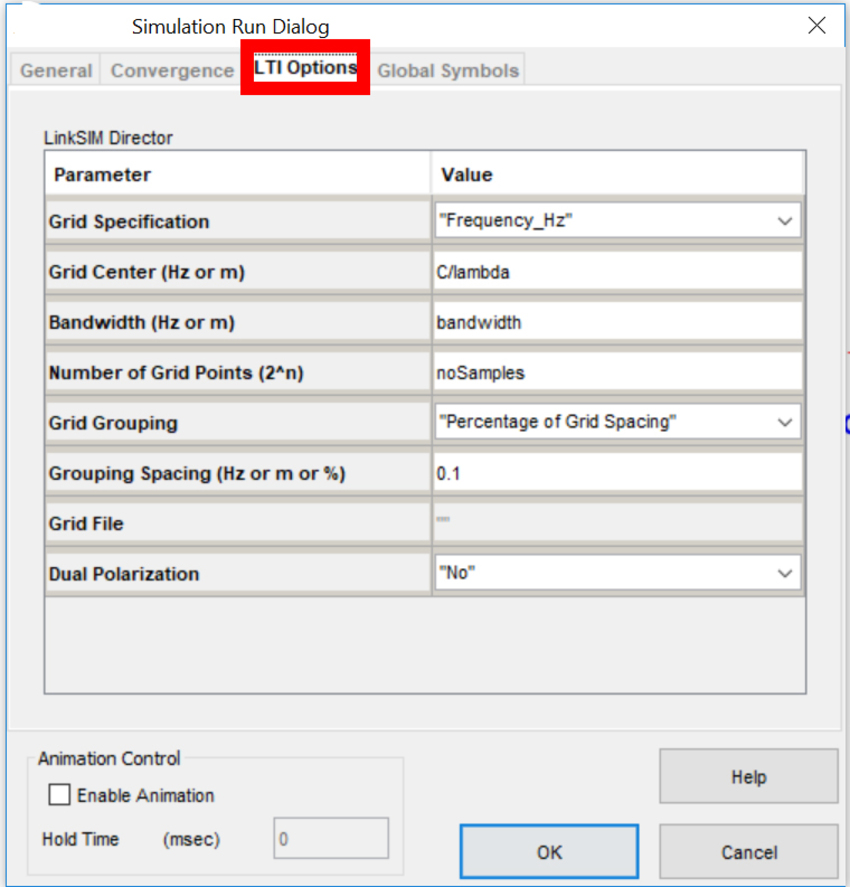Figure 2. Specifying LTI parameters.

## Parameters

• Grid Specification (“Frequency_Hz”, “Wavelength_m”, “File”) – Select one of the options for proper interpretation of some of the following parameters
• Grid Center (Hz or m) – Center frequency for the grid
• Bandwidth (Hz or m) – The LTI bandwidth specified directly rather than requiring bitRate and pointsPerBit in this context. The value is either in Hz or m depending on the “Grid Specification”
• Number of Grid Points (2^n) – The number grid points (power of 2)
• Grid Grouping (“Frequency”, “Wavelength”, “Percent of Grid Spacing”, “None”) If LTI grid needs to be on a coarser grid than the default simulation grid, choose an option other than “none” to indicate if wavelength grouping is used and how it is specified by selecting one of the options
• Grouping Spacing (Hz or m or %) – The wavelength spacing based on the “Grid Grouping” above and the “Grid Specification”
• Grid File – Allows you to browse and select a file with the grid specification. The data file header line (the first line in the data file) should be as follows with one of the following choices for the frequency unit
•                 LTIGridFormat1 [nm] | [um]| [m] | [THz] | [Hz]

The subsequent lines of the data file list one frequency or wavelength value per line.

• Dual Polarization (“Yes”, “No”) – Whether the LTI solution is obtained for X-polarization only, or both.

## Test Cases

As an example of a circuit under test, we shall consider the schematic of the design file named:First, we shall run the example without using LTI engine. Next, we shall use LTI.

The schematic (Fig. 3) comprises of 25 coupled ring resonators with a 2-channel wavelength division multiplexed (WDM) input.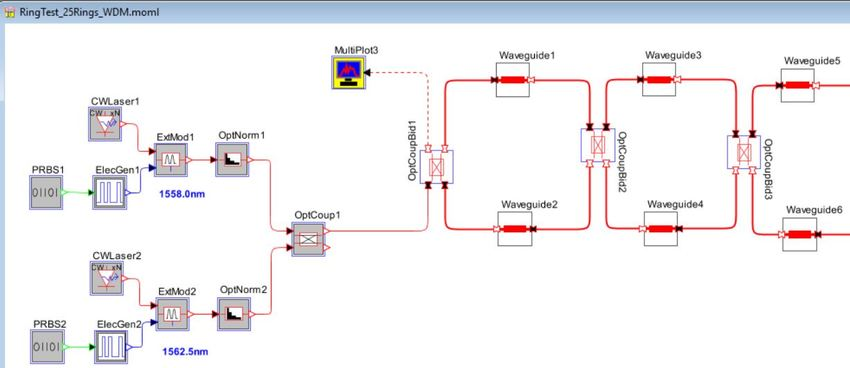Figure 3. Part of the schematic for 25 coupled ring resonators.

To run the simulation, click on the “GO” button. Time-sampled optical 2-channel WDM signal is propagated through the circuit and bidirectional interactions are taken into account. In this simulation, we did not use LTI engine. The simulation takes around 35 minutes (may vary depending on the available computational resources). The transmitted and reflected spectra are shown in Figure 4.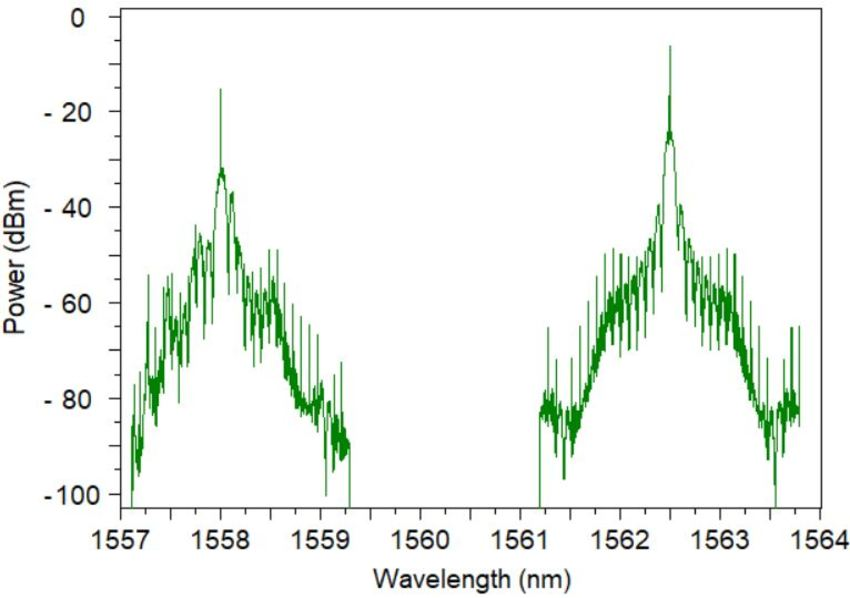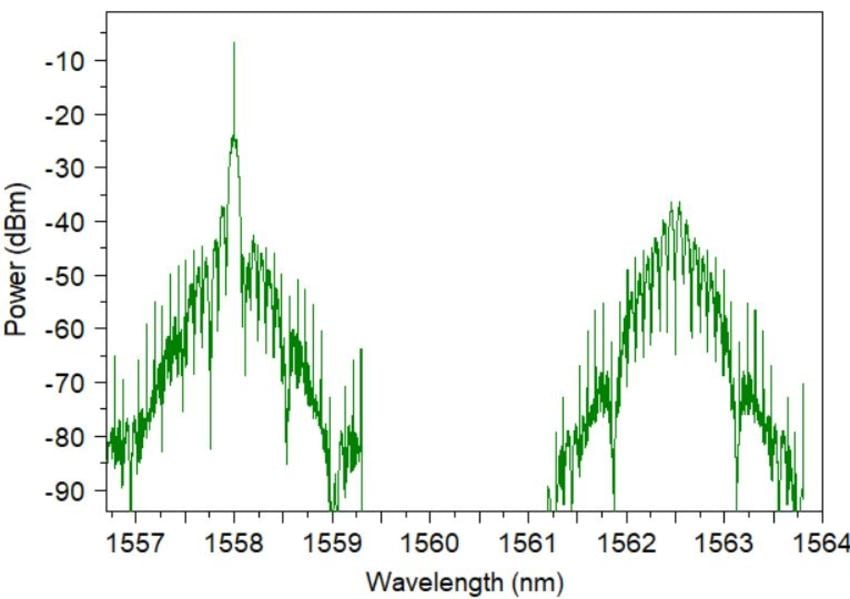Figure 4. Transmitted (left) and reflected (right) spectra for the schematic in Figure 3.

Next, we run the same simulation but this time using LTI engine. The design file:is the LTI compound component with 25 coupled ring resonators, shown in Figure 5.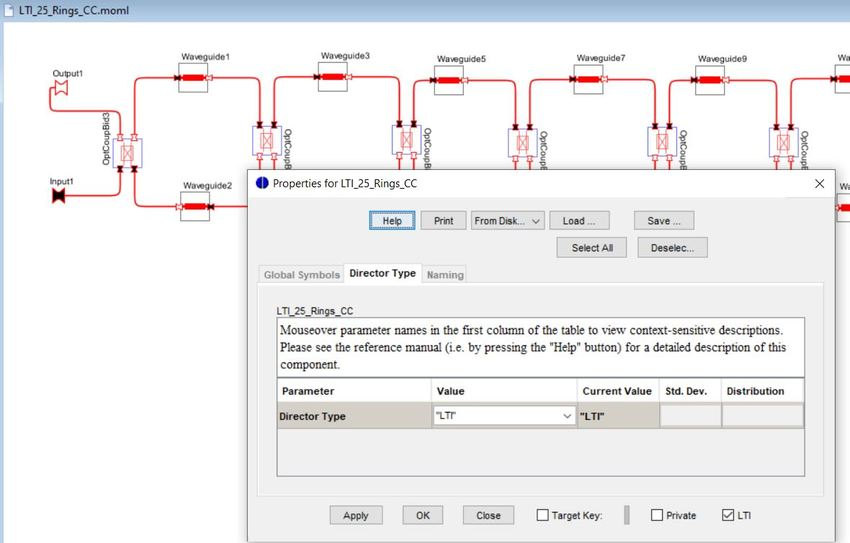Figure 5. Part of the schematic for 25 coupled ring resonators.

Figure 6 shows the main project file:with two WDM lasers driving the LTI CC of Figure 5.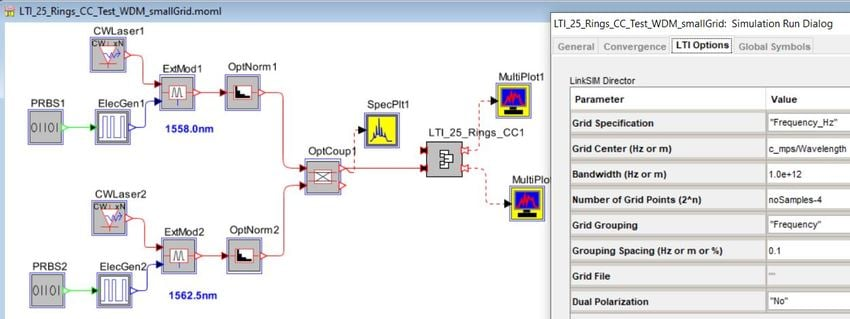Figure 6. LTI simulation setup of the schematic with 25 coupled ring resonators.

To run the LTI simulation, click on the “GO” button, click on the “LTI Options” tab to verify/setup LTI grid options, and click on “OK.” The LTI simulation is carried out on the circuit inside the LTI CC and the time-domain response is evaluated at the two laser frequencies. The simulation takes less than a minute and the results are the same as in Fig. 4.

In this illustration, we observed 35 times speed improvement in simulation time by using LTI simulation engine of OptSim Circuit. Time savings can be even more significant in cases with large parametric and Monte Carlo scans over LTI circuits.

## LTI - Frequently Asked Questions

As an aid to the designer, below is a list of frequently asked questions and answers.

• What if I place a non-LTI component inside an LTI CC?

You’ll get an error message at run time

• What if I place an analyzer inside an LTI CC?

You’ll get an error message at run time

• Can I have PDKs inside LTI CC?

Yes, as long as they don’t have any non-LTI block

• Can I have models/PDKs with data files (say, parametric PDKs) inside LTI CC?

Yes, as long as they don’t have any non-LTI block

• Can I load LTI CC as “in-line compound component?”

Yes, both as “regular” and as “in-line” compound components are allowed

• Can an LTI CC contain another LTI CC inside?

No; currently this is not allowed

• Can a testbed schematic (i.e., the main design file that uses LTI CC) contain more than one LTI CC?

Yes; they all will be simulated on a common LTI grid (i.e., no individual LTI grid supported)

• Can two LTI CCs be directly connected to each other in a testbed schematic?

No, they should be merged into a single LTI CC; or, you can get around this restriction by having an attenuator model with 0dB gain between two LTI CCs

• Since LTI is solved in frequency domain, can I provide a time-domain stimulus to the LTI CC and obtain a time-domain output? If I can, do I also get LTI speed improvement?

“Yes” to both questions. The current tutorial considered this case.

• What should be my number of LTI grid points?

There’s no general answer as it depends on the spectral characteristics of the LTI. If the grid spacing is coarse (in terms of phase variations), the phase interpolation may not be accurate - use grid grouping if that’s the case (more on it, below)

• When should I use LTI grid grouping?

Grid grouping should be done if (a) the LTI S-matrix is calculated on a grid different from the input signal simulation grid and (b) if the grid spacing is coarse enough that phase interpolation may not be accurate. If you simulate on the exact same grid as the input signals, you’ll never do interpolation and so grid grouping should not be needed

• How can I convert an existing CC with only passive components to an LTI CC?

To convert an existing CC containing only passives to an LTI CC, open the existing CC, check “LTI” from the properties dialog window and save the new LTI CC.

## Conclusion

This tutorial demonstrated significant productivity gains from the LTI simulation engine of OptSim Circuit. The tutorial also summarized commonly asked questions and answers in setting up an LTI simulation. Please contact photonics_support@synopsys.com in case of questions or for more information on your modeling needs.